# Research Interests

• Root-finding
• Matrix Polynomials
• Matrix Functions
• Canonical Forms
• Complex Network Analysis
• Theory of numerical condition
• Mathematical analysis of algorithm stability
• Design of numerical algorithms
• Applications of network analysis to science (e.g. biology, economics, finance)
• Applications of matrix theory to pure mathematics (e.g. algebraic topology, group theory, number theory)
• ## Publications

Items labelled by "u" are unpublished in peer reviewed journals (they may be currently under the refereeing process).

7u. Computing a compact local Smith form, with P. Van Dooren. Preprint.

6u. Invertible bases and root vectors for analytic matrix-valued functions. Preprint.

5u. The DL(P) vector space of pencils for singular matrix polynomials, with F. Dopico. Preprint.

4u. Eigenvalue avoidance of structured matrices depending smoothly on a real parameter, with Y. Nakatsukasa. Preprint.

3u. Weighted enumeration of nonbacktracking walks on weighted graphs, with F. Arrigo, D. J. Higham and R. Wood. Preprint.

2u. Tropical Laurent series, their tropical roots, and localization results for the eigenvalues of nonlinear matrix functions, with G. M. Negri-Porzio and L. Robol. Preprint.

1u. On the Jordan form of the Fréchet derivative of a matrix function.

45. Perturbation theory of transfer function matrices, with L. Nyman, J. Pérez and M. C. Quintana. Preprint. SIAM Journal on Matrix Analysis and Applications, To appear, 2023.

44. On the Rellich eigendecomposition of para-Hermitian matrices and the sign characteristics of *-palindromic matrix polynomials, with G. Barbarino. Preprint. Linear Algebra and its Applications, Vol. 672, pp. 1--27, 2023.

43. Revisiting the matrix polynomial greatest common divisor, with P. Van Dooren. Preprint. SIAM Journal on Matrix Analysis and Applications, To appear, 2023.

42. When can forward stable algorithms be composed stably?, with C. Beltrán and N. Vannieuwenhoven. Preprint. IMA Journal of Numerical Analysis, To appear, 2023.

41. On computing root polynomials and minimal bases of matrix pencils, with P. Van Dooren. Preprint. Linear Algebra and its Applications, Vol. 658, pp. 86--115, 2023.

40. Root vectors of polynomial and rational matrices: theory and computation, with P. Van Dooren. Preprint. Linear Algebra and its Applications, Vol. 656, pp. 510--540, 2023.

39. On role extraction for digraphs via neighborhood pattern similarity, with G. Barbarino and P. Van Dooren. Preprint. Physical Review E, Vol. 106, 054301, 2022.

38. Dynamic Katz and related network measures, with F. Arrigo, D. J. Higham and R. Wood. Preprint. Linear Algebra and its Applications, Vol. 655, pp. 159--185, 2022.

37. Cyclically presented groups as Labelled Oriented Graph groups, with G. Williams. Preprint. Journal of Algebra, Vol. 605, pp. 179--198, 2022.

36. The limit empirical spectral distribution of Gaussian monic complex matrix polynomials, with G. Barbarino. Preprint. Journal of Theoretical Probability, 2022, https://doi.org/10.1007/s10959-022-01163-3.

35. The limit empirical spectral distribution of complex matrix polynomials, with G. Barbarino. Preprint. Random Matrices: Theory and Applications, 2250023, 2021.

34. Matrices in companion rings, Smith forms, and the homology of 3-dimensional Brieskorn manifolds, with G. Williams. Preprint. Journal of Algebra, Vol. 587, pp. 1--19, 2021.

33. Nearest Ω-stable matrix via Riemannian optimization, with F. Poloni. Preprint. Numerische Mathematik, Vol. 148, pp. 817--851, 2021.

32. A theory for backtrack-downweighted walks, with F. Arrigo and D. J. Higham. Preprint. SIAM Journal on Matrix Analysis and Applications, Vol. 42, No.3, pp. 1229--1247, 2021.

31. Structured backward errors in linearizations, with L. Robol and R. Vandebril. Preprint. Electronic Transactions on Numerical Analysis, Vol. 54, pp. 420--442, 2021.

30. Beyond non-backtracking: non-cycling centrality measures, with F. Arrigo and D. J. Higham. Proceedings of the Royal Society A, Vol. 476:20190653, 2020.

29. Wilkinson's bus: Weak condition numbers, with an application to singular polynomial eigenproblems, with M. Lotz. Preprint. Foundations of Computational Mathematics, Vol. 20, pp. 1439--1473, 2020.

28. Root polynomials and their role in the theory of matrix polynomials, with F. Dopico. Preprint. Linear Algebra and its Applications, Vol. 584, pp. 37-78, 2020.

27. Non-backtracking PageRank, with F. Arrigo and D. J. Higham. Journal of Scientific Computing, Vol. 80, No.3, pp. 1419-1437, 2019.

26. The structured condition number of a differentiable map between matrix manifolds, with applications, with B. Arslan and F. Tisseur. Preprint. SIAM Journal on Matrix Analysis and Applications, Vol. 40, No. 2, pp. 774-799, 2019.

25. Inertia laws and localization of real eigenvalues for generalized indefinite eigenvalue problems , with Y. Nakatsukasa. Preprint. Linear Algebra and its Applications, Vol. 578, pp. 272-296, 2019.

24. Non-backtracking alternating walks, with F. Arrigo and D. J. Higham. Preprint. SIAM Journal on Applied Mathematics, Vol. 79, No. 3, pp. 781-801, 2019.

23. On the exponential generating function for non-backtracking walks, with F. Arrigo, P. Grindrod and D. J. Higham. Preprint. Linear Algebra and its Applications, Vol. 556, pp. 381-399, 2018.

22. Nonbacktracking walk centrality for directed networks, with F. Arrigo, P. Grindrod and D. J. Higham. Preprint. Journal of Complex Networks, Vol. 6, No.1, pp. 54-78, 2018.

21. The deformed graph Laplacian and its applications to network centrality analysis, with P. Grindrod and D. J. Higham. Preprint. SIAM Journal on Matrix Analysis and Applications, Vol. 39, No. 1, pp. 310-341, 2018.

20. A formula for the Fréchet derivative of a generalized matrix function. Preprint. SIAM Journal on Matrix Analysis and Applications, Vol. 38, No.2, pp. 434-457, 2017.

19. Vector spaces of linearizations of matrix polynomials: a bivariate polynomial approach, with Y. Nakatsukasa and A. Townsend. Preprint. SIAM Journal on Matrix Analysis and Applications, Vol. 38, No. 1, pp. 1-29, 2017.

18. Chebyshev rootfinding via computing eigenvalues of colleague matrices: when is it stable?, with J. Pérez. Preprint. Mathematics of Computation, Vol. 86, No. 306, pp. 1741-1767, 2017.

17. On the sign characteristics of Hermitian matrix polynomials, with V. Mehrmann, F.Tisseur, and H. Xu. Preprint. Linear Algebra and its Applications, Vol. 511, pp. 328-364, 2016.

16. Fiedler-comrade and Fiedler-Chebyshev pencils, with J. Pérez. Preprint. SIAM Journal on Matrix Analysis and Applications, Vol. 37, No. 4, pp. 1600-1624, 2016.

15. On the stability of computing polynomial roots via confederate linearizations, with Y. Nakatsukasa. Preprint. Mathematics of Computation, Vol. 85, No. 301, pp. 2391-2425, 2016.

14. An algorithm to compute the polar decomposition of a 3x3 matrix, with N. J. Higham. Preprint. Numerical Algorithms, Vol. 73, No. 2, pp. 349-369, 2016.

13. Numerical instability of resultant methods for multidimensional rootfinding, with A. Townsend. Preprint. SIAM Journal on Numerical Analysis, Vol. 54, No. 2, pp. 719-743, 2016.

12. When is a Hamiltonian matrix the commutator of two skew-Hamiltonian matrices? Preprint. Linear and Multilinear Algebra, Vol. 63, No. 8, pp. 1531-1552, 2015.

11. Duality of matrix pencils, Wong chains and linearizations, with F. Poloni. Preprint. Linear Algebra and its Applications, Vol. 471, pp 730-767, 2015.

10. Tropical roots as approximations to eigenvalues of matrix polynomials, with M. Sharify and F. Tisseur. Preprint. SIAM Journal on Matrix Analysis and Applications, Vol. 36, No. 1, pp. 138-157, 2015.

9. Computing the common zeros of two bivariate functions via Bézout resultants , with Y. Nakatsukasa and A. Townsend. Preprint. Numerische Mathematik, Vol. 129, No. 1, pp.181-209, 2015.

8. Flanders' theorem for many matrices under commutativity assumptions, with F. De Terán, R. Lippert and Y. Nakatsukasa. Preprint. Linear Algebra and its Applications, Vol. 443, pp. 120-138, 2014.

7. Locating the eigenvalues of matrix polynomials, with D. A. Bini and M. Sharify. Preprint. SIAM Journal on Matrix Analysis and Applications, Vol. 34, No. 4, pp. 1708-1727, 2013.

6. Solving polynomial eigenvalue problems by means of the Ehrlich-Aberth method, with D. A. Bini. Preprint. Linear Algebra and its Applications, Vol. 439, No. 4, pp. 1130-1149, 2013.

5. The Ehrlich-Aberth method for palindromic matrix polynomials represented in the Dickson basis, with L. Gemignani. Linear Algebra and its Applications, Vol. 438, No.4, pp. 1645-1666, 2013.

4. Modifications of Newton's method for even-grade palindromic polynomials and other twined polynomials, with L. Gemignani. Numerical Algorithms, Vol. 61, No.2, pp. 315-329, 2012.

3. The behaviour of the complete eigenstructure of a polynomial matrix under a generic rational transformation . Preprint. Electronic Journal of Linear Algebra, Vol. 23, pp. 607-624, 2012.

2. The application of the Ehrlich-Aberth method to structured polynomial eigenvalue problems . Link. PAMM, Vol. 11, No.1, pp. 919-922, 2011.

1. Algebraic arctic curves in the domain-wall six-vertex model, with F. Colomo and A. G. Pronko. Preprint. Journal of Physics A: Mathematical and Theoretical, Vol. 44, No.19, 195201, 2011.

## Software

7. V. Noferini and P. Van Dooren, compactSmith, a function to compute the ``compact" local Smith form. MATLAB implementation available via github . 2023.

5. V. Noferini and P. Van Dooren, GCRDr, a function to compute the compact greatest common right divisor of a pair of polynomial matrices having the same number of columns. MATLAB implementation available via github . 2022.

5. V. Noferini and F. Poloni, nearest_real_eigenvalues, a function to compute the nearest matrix with all real eigenvalues. MATLAB implementation available via github . 2020.

4. V. Noferini and F. Poloni, nearest_stable, two functions to compute the nearest (real or complex: available are different codes for each version) Hurwitz stable matrix. MATLAB implementation available via github . 2020.

3. N. J. Higham and V. Noferini, polar_quaternion, a function to stably compute the polar decomposition of a 3x3 real matrix via quaternions. MATLAB implementation available via github . 2017.

2. Y. Nakatsukasa, V. Noferini and A. Townsend, rootsb, a function to compute the common real zeros of two bivariate functions. Available in MATLAB Central File Exchange. 2013.

1. Y. Nakatsukasa, V. Noferini and A. Townsend, chebfun2/roots, a function to compute the common real zeros of two bivariate functions. Available within the MATLAB package chebfun. 2013.

## Coauthors

(includes papers in preparation not yet listed above)
 Francesca Arrigo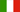Bahar Arslan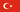Giovanni BarbarinoCarlos Beltrán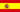Dario A. BiniFilippo ColomoFernando De Terán V.Froilán M. DopicoLuca GemignaniPeter Grindrod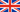Desmond J. HighamNicholas J. HighamRoss Lippert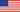Martin Lotz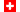Volker Mehrmann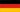Yuji Nakatsukasa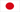Gian M. Negri-PorzioLauri Nyman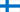Javier Pérez A.Federico PoloniAndrei G. Pronko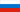María C. Quintana P.Leonardo RobolMeisam Sharify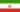Françoise Tisseur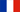Alex TownsendRaf Vandebril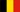Paul Van DoorenNick VannieuwenhovenSpyridon Vrontos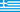Gerald WilliamsRyan WoodHongguo Xu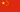Ion Zaballa## A nondecreasing integer sequence

Number of unique visitors of this page since May 14th, 2015